# High School Math : Taylor and Maclaurin Series

## Example Questions

### Example Question #1 : Understanding Taylor Series

Give theterm of the Maclaurin series of the function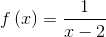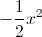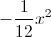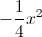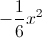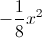Explanation:

Theterm of the Maclaurin series of a functionhas coefficient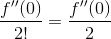The second derivative ofcan be found as follows: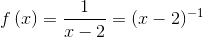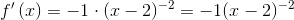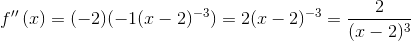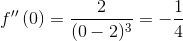The coeficient ofin the Maclaurin series is therefore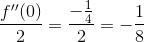### Example Question #2 : Understanding Taylor Series

Give the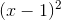term of the Taylor series expansion of the function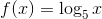about.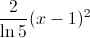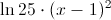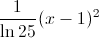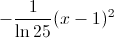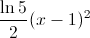Explanation:

Theterm of a Taylor series expansion aboutis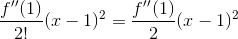.

We can find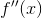by differentiating twice in succession: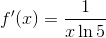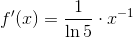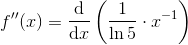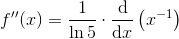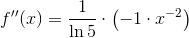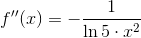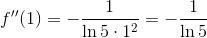so theterm is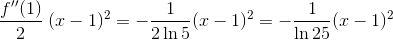### Example Question #1 : Taylor And Maclaurin Series

Give the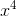term of the Maclaurin series expansion of the function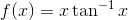.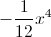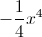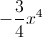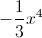Explanation:

This can most easily be answered by recalling that the Maclaurin series for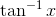is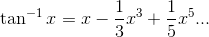Multiply byto get: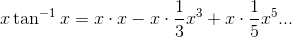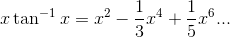Theterm is therefore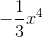.

### Example Question #2 : Understanding Maclaurin Series

Give the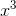term of the Maclaurin series of the function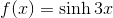.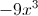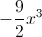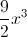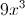Explanation:

Theterm of a Maclaurin series expansion has coefficient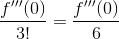.

We can find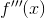by differentiating three times in succession: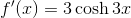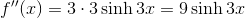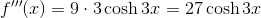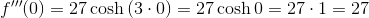The term we want is therefore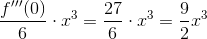### Example Question #1 : Applying Taylor Series

Give theterm of the Maclaurin series expansion of the function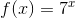.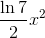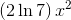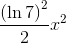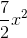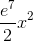Explanation:

Theterm of a Maclaurin series expansion has coefficient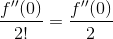.

We can findby differentiating twice in succession: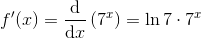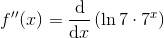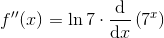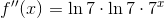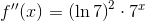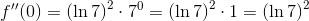The coefficient we want is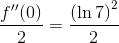,

so the corresponding term is.